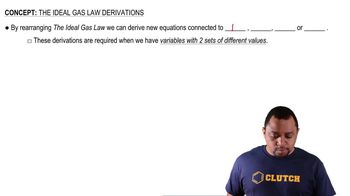Start typing, then use the up and down arrows to select an option from the list.
2:45 minutes
Problem 98
Textbook Question

# Consider a lake that is about 40 m deep. A gas bubble with a diameter of 1.0 mm originates at the bottom of a lake where the pressure is 405.3 kPa. Calculate its volume when the bubble reaches the surface of the lake where the pressure is 98 kPa, assuming that the temperature does not change.Verified Solution
This video solution was recommended by our tutors as helpful for the problem above.
94views